Next: The order Up: Implementation Previous: Type of the functions   Contents

Interval Function

The user must provide a function which will compute the function intervals of the functions for a given box. When designing ALIAS we have determined that to be efficient we need a procedure that allow to calculate the interval evaluation of all the functions or only a subgroup of them in order to avoid unnecessary calculations. Hence the syntax of this procedure is:

INTERVAL_VECTOR IntervalFunction (int l1,int l2,INTERVAL_VECTOR & x)
• x: a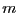dimensional interval vector which define the intervals for the unknowns
• l1,l2: the function must be able to return the interval value of the functions l1 to l2. The first function has number 1, the last m. So if l1=l2=1 the function should return an interval vector whose only the first component has been computed.
This function should be written using the BIAS/Profil rules. If you have equations and inequalities in the system you must define first the equations and then the inequalities.

The efficiency of the algorithm is heavily dependent on the way this procedure is written. Two factors are to be considered:

• efficiency of the evaluation
• sharp bound on the evaluation
Efficiency will enable to decrease the computation time of the evaluation. Let consider for example the following system: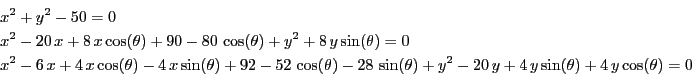The evaluation function may be written as:

e1 = Sqr(x)+Sqr(y)-50.0 ;
e2 =Sqr(x)-20.0*x+8.0*x*Cos(teta)+90.0-80.0*Cos(teta)+Sqr(y)+8.0*y*Sin(teta);
e3 =Sqr(X)-6.0*x+4.0*x*Cos(teta)-4.0*x*Sin(teta)+92.0-52.0*Cos(teta)-28.0*
Sin(teta)+SQR(Y)-20.0*y+4.0*y*Sin(teta)+4.0*y*Cos(teta);
or, using temporary variables:

t1 = Sqr(x);
t2 = Sqr(y);
t5 = Cos(teta);
t6 = x*t5;
t9 = Sin(teta);
t10 = y*t9;
e1 = t1+t2-50.0;
e2 = t1-20.0*x+8.0*t6+90.0-80.0*t5+t2+8.0*t10;
e3 = t1-6.0*x+4.0*t6-4.0*x*t9+92.0-52.0*t5-28.0*t9+t2-20.0*y+4.0*t10+4.0*y*t5;
the second manner is more efficient as the intervals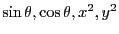,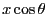,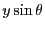are evaluated only once instead of 3 or 2 in the first evaluation. Note also that for speeding up the computation it may be interesting to declare the variables t1, t2, t5, t6, t9, t10 as global to avoid having to create a new interval data structure at each call of the evaluation function.

The second point is the sharpness of the evaluation. Let consider the polynomial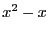. If the variable lie in the interval [0,1] the evaluation will lead to the interval [-1,1]. The same polynomial may we written in Horner form as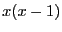the function being then evaluated as [-1,0]. Now suppose that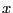lie in [0.8,1.1]. The initial polynomial will be evaluated as [-0.46,0.41] while in Horner form the evaluation leads to [-0.22,0.11]. But this polynomial may also be written as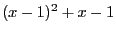(which is the centered form at 1) whose evaluation leads to [-0.2,0.14] which has a sharper lower bound than in the Horner form (note that Horner form is very efficient for the evaluation of a polynomial but do not lead always to the sharpest evaluation of the bounds on the polynomial although this is some time mentioned in the literature). Unfortunately there is no known method which enable to determine what is the best way to express a given function in order to get the sharpest possible bounds. For complex expression you may use the procedures MinimalCout or Code of ALIAS-Maple that try to produce the less costly formulation of a given expression.

Another problem is the cost of the tests which are necessary to determine if the interval evaluation of one of the function does not include 0. Indeed let us assume that we have 40 equations and 7 unknowns and that we are considering a box such that the function interval all contain 0. When testing the functions we may either evaluate all the functions with one procedure call (with the risk of performing useless evaluations e.g. if the interval evaluation of the first equations does not contain 0) or evaluate the functions one after the other (at a cost of 40 procedure calls but avoiding useless equation evaluations). The best way balances the cost of procedure calls compared to the cost of equation evaluations. By default we are evaluating all the functions in one step but by setting the variable Interval_Evaluate_Equation_Alone to 1 the program will evaluate the functions one after the other.

A last problem is the interval valuation of the equations. Indeed you may remember that some expression may not be evaluated for some ranges for the unknowns (see section 2.1.1.3). If such problem may occur a solution is to include into this procedure a test before each expression evaluation that verify if the expression is interval-valuable. If not two cases may occur:

• the expression will never be interval-valuated whatever is the value of one of the unknown in its range (e.g. the expression involves ArcSin(x) and the range for x is [-4,-3])
• the expression may be evaluated for some values of the unknowns in their range (e.g. the expression involves Sqrt(x) while the range for x is [-3,10])
In the first case the interval evaluation of the expression should be set to an interval which does not include 0 (hence the algorithm will discard the box). In the second case the best strategy seems to be to set the interval evaluation of the expression to a very large interval that includes 0 (e.g. [-1e8,1e8]). Note that some filtering strategy such as the one described in section 2.17 may help to avoid some of these problems (in the example this strategy will have determined that Sqrt(x) is interval-valuable only for x in [0,10] and will have automatically set the range for x to this value).Next: The order Up: Implementation Previous: Type of the functions   Contents
Jean-Pierre Merlet 2012-12-20